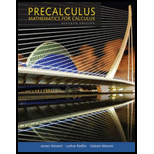Chapter 6.1, Problem 89E### Precalculus: Mathematics for Calcu...

7th Edition
James Stewart + 2 others
ISBN: 9781305071759

#### Solutions

Chapter
Section### Precalculus: Mathematics for Calcu...

7th Edition
James Stewart + 2 others
ISBN: 9781305071759
Textbook Problem

# Speed at the Equator The earth rotates about its axis once every 23 h 56 min 4 s, and the radius of the earth is 3960 mi. Find the linear speed of a point on the equator in mi/h.

To determine

To find: The linear speed of the earth at the equator in mi/h .

Explanation

Given:

The radius of the earth is 3960mi .

Time of rotation of the earth about its axis is 23h56min4s .

Formulas used:

Linear speed is v=st=rθt

Here θ is the angle change in a rotation, s is the distance the point travels in time t and r is the radius of the earth.

Conversion of minutes and seconds to hour

1min=160h1s=13600h

Calculation:

For 1 complete rotation of the earth a circle is covered.

Angle for 1 rotation is 2π .

Covert time into hour.

23h56min4s=23+5660+43600=23+0.934+0.012=23.946h

In 23.946h the earth will rotate about its axis once.

Angle change will be,

θ=1×2π=2π

In 23

### Still sussing out bartleby?

Check out a sample textbook solution.

See a sample solution

#### The Solution to Your Study Problems

Bartleby provides explanations to thousands of textbook problems written by our experts, many with advanced degrees!

Get Started

## Additional Math Solutions

#### Find more solutions based on key concepts